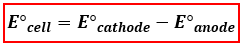# Problem: Nickel and aluminum electrodes are used to build a galvanic cell. The standard reduction potential for the nickel(II) ion is -0.26 V and that of the aluminum(III) ion is -1.66 V.a. What is the theoretical cell potential assuming standard conditions?b. Which metal is the cathode?c. Which statement is true?     - Electrons flow from Ni to Al.     - Electrons flow from Al to Ni.     - The nickel electrode loses mass.     - The aluminum electrode gains mass.d. Type the shorthand notation for this cell. Do not include concentrations.For example, in shorthand notation your answer might look like Cu|Cu + | |Pd2+|Pd

###### FREE Expert Solution

(a) We’re being asked to determine the standard cell potential (E°cellof the reaction occurring in an electrochemical cell. To calculate for the standard cell potential, use the following equation:Determine the anode and cathode by comparing their E° values.

↓ E° → oxidation → anode

↑ E° → reduction → cathode

89% (294 ratings)###### Problem Details

Nickel and aluminum electrodes are used to build a galvanic cell. The standard reduction potential for the nickel(II) ion is -0.26 V and that of the aluminum(III) ion is -1.66 V.

a. What is the theoretical cell potential assuming standard conditions?

b. Which metal is the cathode?

c. Which statement is true?
- Electrons flow from Ni to Al.
- Electrons flow from Al to Ni.
- The nickel electrode loses mass.
- The aluminum electrode gains mass.

d. Type the shorthand notation for this cell. Do not include concentrations.

For example, in shorthand notation your answer might look like Cu|Cu | |Pd2+|Pd